﻿ 凸三角多面体的快速搜索 Fast Searching on Convex Deltahedra

Operations Research and Fuzziology
Vol.08 No.03(2018), Article ID:26459,8 pages
10.12677/ORF.2018.83015

Fast Searching on Convex Deltahedra

Xiaodong Han

College of Math, Physics and Information Technology, Zhejiang Normal University, Jinhua Zhejiang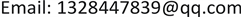Received: Jul. 27th, 2018; accepted: Aug. 9th, 2018; published: Aug. 16th, 2018ABSTRACT

In this paper, we study fast searching graph searching model, which differs from the classical edge searching in one way: every edge is traversed exactly once and searchers are not allowed to jump. The graph of this paper is convex deltahedra. We will examine the fast search number (i.e., the minimum number of searchers required for capturing the fugitive) of all the convex deltahedra. We provide an explicit formula for the fast search number of convex deltahedra.

Keywords:Fast Searching, Graph Searching, Pursuit-Evasion Strategy, Cops and Robber Game1. 引言

1) 放置：某一搜索者被放置到图上的一个顶点；

2) 移动：搜索者从当前顶点沿着一条未被清理过的邻边移动到邻点。

1) 如果搜索者从顶点u移动到顶点v后，顶点u上还至少有一个搜索者；

2) 除边uv以外，顶点u的所有邻边都已被清理。

Dyer和Yang设计了一个线性时间复杂度的图搜索算法用来计算树的快速搜索数，并对二部图的快速搜索数做了相关研究  。2011年Stanley和Yang提出了一个快速搜索数的下界，并设计了计算立方体图和哈林图等的快速搜索数的图搜索算法  。2013年Yang等撰写了关于图搜索及其相关问题的综合性研究论文  。2016年Yang等得出了完全k部图的快速搜索数的一个上界与下界，并对完全二部图和完全分割图这两个特殊的完全k部图做了详细的研究  。2017年Xue和Yang提出了快速搜索的一个新下界，并提出了欧拉图和路径的笛卡尔积图的快速搜索数  。在两个基本模型的基础上，研究人员还提出了混合搜索、快速边搜索等搜索博弈的模型      。

2. 概念

3. 凸三角多面体的快速搜索

2011年Stanley和Yang  提出如下推论和定理：

2011年Yang  提出如下定理：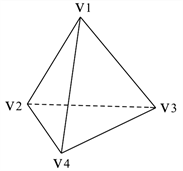Figure 1. Tetrahedron, triangular bipyramid

1) 我们把搜索者 ${\lambda }_{1}$${\lambda }_{2}$${\lambda }_{3}$ 均放置到顶点 ${v}_{1}$ ，把 ${\lambda }_{4}$ 放置到顶点 ${v}_{2}$

2) ${\lambda }_{1}$${\lambda }_{2}$${\lambda }_{3}$ 分别沿边 ${v}_{1}{v}_{2}$${v}_{1}{v}_{3}$${v}_{1}{v}_{4}$ 移动，使得 ${v}_{2}$${v}_{3}$${v}_{4}$ 分别被1个搜索者守卫；

3) ${\lambda }_{4}$${v}_{2}$ 沿环 ${v}_{2}{v}_{3}{v}_{4}{v}_{2}$ 清理回到 ${v}_{2}$

4) ${\lambda }_{1}$${\lambda }_{2}$${\lambda }_{3}$ 分别沿 ${v}_{2}{v}_{5}$${v}_{3}{v}_{5}$${v}_{4}{v}_{5}$ 移动到 ${v}_{5}$ ，完成对图 ${C}_{6}$ 的清理。即 $fs\left({C}_{6}\right)\le 4$

1) 我们把搜索者 ${\lambda }_{1}$${\lambda }_{2}$${\lambda }_{3}$${\lambda }_{4}$ 均放置到顶点 ${v}_{1}$ ，把 ${\lambda }_{5}$ 放置到顶点 ${v}_{2}$

2) ${\lambda }_{1}$${\lambda }_{2}$${\lambda }_{3}$${\lambda }_{4}$ 分别沿边 ${v}_{1}{v}_{2}$${v}_{1}{v}_{3}$${v}_{1}{v}_{4}$${v}_{1}{v}_{5}$ 移动，使得 ${v}_{2}$${v}_{3}$${v}_{4}$${v}_{5}$ 分别被1个搜索者守卫；

3) ${\lambda }_{4}$${v}_{2}$ 沿环 ${v}_{2}{v}_{3}{v}_{4}{v}_{5}{v}_{2}$ 清理回到 ${v}_{2}$

4) ${\lambda }_{1}$${\lambda }_{2}$${\lambda }_{3}$${\lambda }_{4}$ 分别沿 ${v}_{2}{v}_{6}$${v}_{3}{v}_{6}$${v}_{4}{v}_{6}$${v}_{5}{v}_{6}$ 移动到 ${v}_{6}$ ，完成对图 ${C}_{8}$ 的清理。即 $fs\left({C}_{8}\right)\le 5$

1) 我们把搜索者 ${\lambda }_{1}$${\lambda }_{2}$${\lambda }_{3}$${\lambda }_{4}$ 均放置到顶点 ${v}_{2}$ ，把 ${\lambda }_{5}$ 放置到顶点 ${v}_{6}$

2) ${\lambda }_{1}$${\lambda }_{2}$${\lambda }_{3}$${\lambda }_{4}$ 分别沿边 ${v}_{2}{v}_{1}$${v}_{2}{v}_{3}$${v}_{2}{v}_{5}$${v}_{2}{v}_{6}$ 移动，使得 ${v}_{1}$${v}_{3}$${v}_{5}$${v}_{6}$ 分别被至少1个搜索者守卫, ${\lambda }_{5}$${v}_{6}$ 沿环 ${v}_{6}{v}_{4}{v}_{3}{v}_{7}{v}_{6}$ 清理回到 ${v}_{6}$

3) ${\lambda }_{1}$${\lambda }_{3}$ 分别沿 ${v}_{1}{v}_{4}$${v}_{5}{v}_{7}$ 移动，使得 ${v}_{4}$${v}_{7}$ 被一个搜索者守卫；

4) ${\lambda }_{5}$${v}_{6}$ 沿环 ${v}_{6}{v}_{4}{v}_{3}{v}_{7}{v}_{6}$ 清理回到 ${v}_{6}$${v}_{6}$ 上的 ${\lambda }_{3}$ 清理 ${v}_{7}{v}_{4}$ ，完成对图 ${C}_{8}$ 的清理。即 $fs\left({C}_{10}\right)\le 5$

1) 把搜索者 ${\lambda }_{1}$${\lambda }_{2}$${\lambda }_{3}$${\lambda }_{4}$ 均放置到顶点 ${v}_{2}$ ，把 ${\lambda }_{5}$ 放置到顶点 ${v}_{4}$

2) ${\lambda }_{1}$${\lambda }_{2}$${\lambda }_{3}$${\lambda }_{4}$ 分别沿边 ${v}_{2}{v}_{1}$${v}_{2}{v}_{3}$${v}_{2}{v}_{4}$${v}_{2}{v}_{5}$ 移动，使得 ${v}_{1}$${v}_{3}$${v}_{4}$${v}_{5}$ 分别至少被1个搜索者守卫, ${\lambda }_{5}$${v}_{4}$ 沿环 ${v}_{4}{v}_{5}{v}_{3}{v}_{1}{v}_{4}$ 清理回到 ${v}_{4}$

3) ${\lambda }_{1}$${\lambda }_{5}$ 分别沿 ${v}_{1}{v}_{6}$${v}_{4}{v}_{6}$ 移动(此时 ${v}_{6}$ 被2个搜索者守卫)；

4) ${\lambda }_{4}$ 沿 ${v}_{4}{v}_{7}$ 移动到顶点 ${v}_{7}$${v}_{6}$ 上的 ${\lambda }_{5}$ 沿路径 ${v}_{6}{v}_{7}{v}_{5}{v}_{8}$ 移动到 ${v}_{8}$

5) ${\lambda }_{4}$ 沿 ${v}_{7}{v}_{8}$ 移动到 ${v}_{8}$ ，然后 ${v}_{8}$ 上的 ${\lambda }_{5}$ 沿 ${v}_{8}{v}_{3}$ 移动到 ${v}_{3}$

6) ${v}_{3}$ 上的 ${\lambda }_{5}$ 清理 ${v}_{3}{v}_{6}$ ，完成对图 ${C}_{12}$ 的清理。即 $fs\left({C}_{12}\right)\le 5$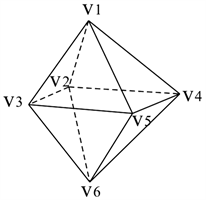Figure 2. Octahedron, pentagonal bipyramid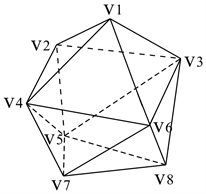Figure 3. Snub disphenoid, triaugmented triangular prism

Case 1：初始顶点的度数为4，不失一般性，我们选取 ${v}_{1}$ 作为初始顶点。

1) 搜索者 ${\lambda }_{1}$${\lambda }_{2}$${\lambda }_{3}$${\lambda }_{4}$ 均放置到顶点 ${v}_{1}$

2) ${\lambda }_{1}$${\lambda }_{2}$${\lambda }_{3}$${\lambda }_{4}$${v}_{1}$ 沿领边进行清理，移动到其4个邻点 ${v}_{2}$${v}_{3}$${v}_{4}$${v}_{6}$ (此时这4个搜索者不满足继续移动清理图的条件而只能守卫)；

3) 不失一般性，放置 ${\lambda }_{5}$${v}_{3}$

4) ${\lambda }_{5}$ 沿环 ${v}_{3}{v}_{2}{v}_{6}{v}_{4}{v}_{3}$ 清理回到 ${v}_{3}$ (此时 ${v}_{3}$ 有两条邻边未被清理且有2个搜索者 ${\lambda }_{2}$${\lambda }_{5}$ )；

5) ${\lambda }_{2}$${\lambda }_{5}$${v}_{3}$ 分别沿邻边进行清理，移动到 ${v}_{8}$${v}_{5}$

Case 2：初始顶点的度数为5，不失一般性，我们选取 ${v}_{2}$ 作为初始顶点。

1) 放置 ${\lambda }_{1}$${\lambda }_{2}$${\lambda }_{3}$${\lambda }_{4}$${\lambda }_{5}$${v}_{2}$ ，然后 ${\lambda }_{1}$${\lambda }_{2}$${\lambda }_{3}$${\lambda }_{4}$${\lambda }_{5}$ 分别沿邻边进行清理，移动到其5个邻点 ${\lambda }_{1}$${\lambda }_{3}$${\lambda }_{4}$${\lambda }_{5}$${\lambda }_{7}$ ，此时5个搜索者所在的顶点上只有1个搜索者且其未被清理的邻边不止一条，因此这5个搜索者不满足继续移动清理图的条件。

2) 我们再放置第6个搜索者 ${\lambda }_{6}$${v}_{6}$ ，然后 ${\lambda }_{6}$${v}_{6}$ 沿路径 ${v}_{6}{v}_{8}{v}_{5}{v}_{2}{v}_{7}$ 清理移动到 ${v}_{7}$

3) ${\lambda }_{4}$${v}_{6}$ 沿邻边 ${v}_{6}{v}_{9}$ 移动到 ${v}_{9}$ ，然后 ${\lambda }_{2}$${v}_{8}$ 沿路径 ${v}_{8}{v}_{9}{v}_{4}{v}_{7}{v}_{5}{v}_{9}{v}_{7}$ 清理，完成对图 ${C}_{14}$ 的清理， 即 $fs\left({C}_{14}\right)\le 6$ 。综上得证： $fs\left({C}_{14}\right)=6$ (图3)。

Case 1：初始顶点的度数为5，不失一般性，我们选取顶点 ${v}_{3}$ 作为初始顶点。

1) 放置 ${\lambda }_{1}$${\lambda }_{2}$${\lambda }_{3}$${\lambda }_{4}$${\lambda }_{5}$${v}_{3}$

2) ${\lambda }_{1}$${\lambda }_{2}$${\lambda }_{3}$${\lambda }_{4}$${\lambda }_{5}$${v}_{3}$ 分别沿邻边进行清理，到其5个邻点 ${v}_{1}$${v}_{2}$${v}_{6}$${v}_{9}$${v}_{4}$ (此时这5个搜索者不满足继续移动清理图的条件而只能守卫)；

3) 放置 ${\lambda }_{6}$ 到顶点度数为4的顶点 ${v}_{1}$ 的邻点 ${v}_{2}$ 处(此时 ${v}_{2}$ 上有2个搜索者 ${\lambda }_{2}$${\lambda }_{6}$ )；

4) ${\lambda }_{6}$ 沿环 ${v}_{2}{v}_{6}{v}_{9}{v}_{4}{v}_{1}{v}_{2}$ 清理回到 ${v}_{2}$ (此时 ${v}_{1}$ 有一条邻边未被清理且有1个搜索者 ${\lambda }_{1}$ )；

5) ${\lambda }_{1}$ 沿着邻边 ${v}_{1}{v}_{5}$${v}_{5}$${\lambda }_{2}$ 沿邻边 ${v}_{2}{v}_{5}$${v}_{5}$ (此时 ${v}_{2}$ 有一条邻边未被清理且有1个搜索者 ${\lambda }_{6}$${v}_{5}$ 上有2个搜索者 ${\lambda }_{1}$${\lambda }_{2}$ )；

6) ${\lambda }_{6}$ 沿邻边 ${v}_{2}{v}_{7}$${v}_{7}$

(A) 选择有两条邻边未被清理的顶点

a) ${\lambda }_{2}$ 沿 ${v}_{5}{v}_{4}$ 移动到 ${v}_{4}$ (此时 ${v}_{4}$ 上有2个搜索者 ${\lambda }_{2}$${\lambda }_{5}$ ，且只有一条邻边 ${v}_{4}{v}_{8}$ 未被清理)；

b) ${\lambda }_{2}$ 沿 ${v}_{4}{v}_{8}$ 移动到 ${v}_{8}$

(B) 选择有三条邻边未被清理的顶点

a) ${\lambda }_{2}$ 沿 ${v}_{5}{v}_{7}$ 移动到 ${v}_{7}$ ，此时 ${v}_{7}$ 上有2个搜索者 ${\lambda }_{2}$${\lambda }_{6}$ ，可以移动其中1个；但是有三条邻边 ${v}_{7}{v}_{6}$${v}_{7}{v}_{8}$${v}_{7}{v}_{10}$ 未被清理，此时不满足继续移动清理图的条件；

b) 如果 ${\lambda }_{6}$ 沿边 ${v}_{7}{v}_{8}$ 移动到 ${v}_{8}$ ，由于此时 ${v}_{8}$ 上1个搜索者且四条邻边，此时不满足继续移动清理图的条件；

c) 如果 ${\lambda }_{6}$ 沿边 ${v}_{7}{v}_{10}$ 移动到 ${v}_{10}$ ，由于此时 ${v}_{10}$ 上1个搜索者且四条邻边，此时不满足继续移动清理图的条件；

i) 如果 ${\lambda }_{6}$ 沿 ${v}_{7}{v}_{6}$ 移动到 ${v}_{6}$ (此时 ${v}_{6}$ 有2个搜索者 ${\lambda }_{3}$${\lambda }_{6}$ ，可以移动其中1个；且只有一条邻边 ${v}_{6}{v}_{10}$ 未被清理)；

ii) ${\lambda }_{3}$ 沿 ${v}_{6}{v}_{10}$ 移动到 ${v}_{10}$

Case 2：初始顶点的度数为4，不失一般性，我们选取顶点 ${v}_{1}$ 作为初始顶点。

1) 放置 ${\lambda }_{1}$${\lambda }_{2}$${\lambda }_{3}$${\lambda }_{4}$${v}_{1}$ ；然后 ${\lambda }_{1}$${\lambda }_{2}$${\lambda }_{3}$${\lambda }_{4}$${v}_{1}$ 分别沿邻边进行清理，到其四个邻点 ${v}_{2}$${v}_{3}$${v}_{4}$${v}_{5}$ ，此时这4个搜索者只能守卫而不能再移动；

2) 不失一般性，为了使清理继续下去，放置 ${\lambda }_{5}$ 到顶点 ${v}_{2}$

3) ${\lambda }_{5}$ 沿环 ${v}_{2}{v}_{3}{v}_{4}{v}_{5}{v}_{2}$ 清理回到 ${v}_{2}$ (此时 ${v}_{2}$ 上上有2个搜索者 ${\lambda }_{1}$${\lambda }_{5}$ 且有两条未被清理的边)；

4) ${v}_{2}$ 上的 ${\lambda }_{1}$${\lambda }_{5}$ 沿邻边 ${v}_{2}{v}_{7}$${v}_{2}{v}_{6}$ 到移动 ${v}_{7}$${v}_{6}$

5) 为了使清理继续下去，我们需要放置第6个搜索者 ${\lambda }_{6}$ 到图中，根据放置顶点处，未被清理边的个数，可以分为以下3种情形：

(A) 不失一般性，放置 ${\lambda }_{6}$${v}_{5}$ (此时 ${v}_{5}$ 上有2个搜索者 ${\lambda }_{4}$${\lambda }_{6}$ )；

a) ${\lambda }_{4}$${\lambda }_{6}$ 移动到其邻点 ${v}_{7}$${v}_{8}$ (此时 ${v}_{7}$ 上有2个搜索者 ${\lambda }_{1}$${\lambda }_{4}$ )；

b) 移动 ${\lambda }_{4}$${v}_{10}$ ，此时 ${v}_{10}$ 上只有1个搜索者，清理不能继续下去；

c) 移动 ${\lambda }_{4}$${v}_{8}$ ，此时 ${v}_{8}$ 上有2个搜索者 ${\lambda }_{4}$${\lambda }_{6}$ ，移动 ${\lambda }_{6}$ 到其一个邻点 ${v}_{4}$ ，再移动 ${\lambda }_{6}$${v}_{4}$ 一个邻点 ${v}_{9}$ ，此时 ${v}_{3}$${v}_{6}$${v}_{7}$${v}_{8}$${v}_{9}$ 上有且只有1个搜索者且不止一条未被清理的邻边；显然此时这6个搜索者只能守卫而不能再移动，清理不能继续下去；

(B) 放置 ${\lambda }_{6}$ 到有四条邻边未被清理的顶点上；类上同证!

(C) 放置 ${\lambda }_{6}$ 到有五条邻边未被清理的顶点上；类上同证!

1) 不失一般性，放置搜索者 ${\lambda }_{1}$${\lambda }_{2}$${\lambda }_{3}$${\lambda }_{4}$${\lambda }_{5}$ 到顶点 ${v}_{1}$ ，然后 ${\lambda }_{1}$${\lambda }_{2}$${\lambda }_{3}$${\lambda }_{4}$${\lambda }_{5}$ 分别沿着其邻边移动到 ${v}_{2}$${v}_{3}$${v}_{4}$${v}_{5}$${v}_{6}$ (此时显然不满足继续移动清理的条件)；

2) 分别放置 ${\lambda }_{6}$${\lambda }_{7}$${\lambda }_{8}$${v}_{2}$${v}_{3}$${v}_{4}$ 上；

3) 移动 ${\lambda }_{1}$${\lambda }_{6}$ 分别到 ${v}_{7}$${v}_{8}$ ，移动 ${\lambda }_{2}$${\lambda }_{7}$ 分别到 ${v}_{8}$${v}_{9}$ ，移动 ${\lambda }_{3}$${\lambda }_{8}$ 分别到 ${v}_{9}$${v}_{10}$ (此时 ${v}_{8}$ 上有2个搜索者 ${\lambda }_{6}$${\lambda }_{2}$ ，三条未被清理的邻边； ${v}_{9}$ 上有2个搜索者 ${\lambda }_{7}$${\lambda }_{8}$ ，三条未被清理的邻边)；

4) ${\lambda }_{6}$${\lambda }_{7}$ 分别沿 ${v}_{8}{v}_{12}$${v}_{9}{v}_{12}$ 移动到 ${v}_{12}$ ，然后 ${\lambda }_{6}$ 分别沿 ${v}_{12}{v}_{11}$ 移动到 ${v}_{11}$

4. 总结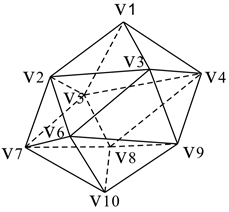Figure 4. Gyroelongated square bipyramid, icosahedron

Fast Searching on Convex Deltahedra[J]. 运筹与模糊学, 2018, 08(03): 119-126. https://doi.org/10.12677/ORF.2018.83015

1. 1. Breisch, R. (1967) An Intuitive Approach to Speleotopology. Southwestern Cavers, 5, 72-78.

2. 2. Parsons, T.D. (1978) Pursuit-Evasion in a Graph. Theory and Applications of Graphs, 642, 426-441. https://doi.org/10.1007/BFb0070400

3. 3. Kirousis, L.M. and Papadimitriou, C.H. (1986) Searching and Pebbling. Theoretical Computer Science, 47, 205-218. https://doi.org/10.1016/0304-3975(86)90146-5

4. 4. Megiddo, N., Hakimi, S.L., Garey, M.R., Johnson, D.S. and Papadimitriou, C.H. (1988) The Complexity of Searching a Graph. Association for Computing Machinery, 35, 18-44. https://doi.org/10.1145/42267.42268

5. 5. Dyer, D., Yang, B. and Yaşar, Ö. (2008) On the Fast Searching Prob-lem. AAIM 2008, LNCS, 5034, 143-154. https://doi.org/10.1007/978-3-540-68880-8_15

6. 6. Stanley, D. and Yang, B. (2011) Fast Searching Games on Graphs. Journal of Combinatorial Optimization, 22, 763-777. https://doi.org/10.1007/s10878-010-9328-4

7. 7. Yang, B. (2013) Fast-Mixed Searching and Related Problems on Graph. Theoretical Computer Science, 507, 100-113. https://doi.org/10.1016/j.tcs.2013.04.015

8. 8. Xue, Y., Yang, B., Zhong, F. and Zilles, S. (2016) Fast Searching on Complete k-Partite Graphs. Conference on Combinatorial Optimization and Applications, 10043, 159-174. https://doi.org/10.1007/978-3-319-48749-6_12

9. 9. Xue, Y. and Yang, B. (2017) The Fast Search Number of a Cartesian Product of Graphs. Discrete Applied Mathematics. https://doi.org/10.1016/j.dam.2017.03.003

10. 10. Dendris, N.D., Kirousis, L.M. and Thilikos, D.M. (1995) Fugi-tive-Search Games on Graphs and Related Parameters. Graph-Theoretic Concepts in Computer Science. Springer, Berlin, Heidelberg. https://doi.org/10.1007/3-540-59071-4_59

11. 11. Yang, B. and Cao, Y. (2008) Monotonicity in Digraph Search Problems. Theoretical Computer Science, 407, 532-544. https://doi.org/10.1016/j.tcs.2008.08.025

12. 12. Richerby, D. and Thilikos, D.M. (2007) Graph Searching in a Crime Wave. International Conference on Graph-Theoretic Concepts in Computer Science, 4769, 21-32. https://doi.org/10.1007/978-3-540-74839-7_3b

13. 13. Yang, B. (2011) Fast Edge Searching and Fast Searching on Graphs. Theoretical Computer Science, 412, 1208-1219. https://doi.org/10.1016/j.tcs.2010.12.065

14. 14. Bonato, A. and Yang, B. (2013) Graph Searching and Related Problems. Handbook of Combinatorial Optimization, 1511-1558. https://doi.org/10.1007/978-1-4419-7997-1_76

15. 15. Bienstock, D. and Seymour, P. (1991) Monotonicity in Graph Searching. Academic Press, Inc., 239-245.

16. 16. Lapaugh, A.S. (1993) Recontamination Does Not Help to Search a Graph. Journal of the ACM, 40, 224-245. https://doi.org/10.1145/151261.151263

17. 17. Yang, B., Dyer, D. and Alspach, B. (2009) Sweeping Graphs with Large Clique Number. Elsevier Science Publishers B. V.

18. 18. Flocchini, P., Fraigniaud, P. and Santoro, N. (2002) Capture of an Intruder by Mobile Agents. ACM, 200-209.

19. 19. Kinnersley, N.G. (1992) The Vertex Separation Number of a Graph Equals Its Path-Width. Information Processing Letters, 42, 345-350. https://doi.org/10.1016/0020-0190(92)90234-M

20. 20. Isaza, A., Lu, J., Bulitko, V., et al. (2008) A Cover-Based Approach to Multi-Agent Moving Target Pursuit. Artificial Intelligence and Interactive Digital Entertainment Conference, Stanford, California, 22-24 October 2008, 54-59.

21. 21. Moldenhauer, C. and Sturtevant, N.R. (2009) Evaluating Strategies for Running from the Cops. International Joint Conference on Artificial Intelligence, Morgan Kaufmann Publishers Inc., 584-589.